# Best Nuclear Physics Books | Develop Application in Nuclear Power

Nuclear Physics covers many important topics that have applications in nuclear physics, nuclear weapons, nuclear medicine, magnetic resonance imaging, industrial and agricultural isotopes, ion implantation in a material engineering field. Here you will get the best nuclear physics books.

## 1. Nuclear Physics for Babies (Baby University)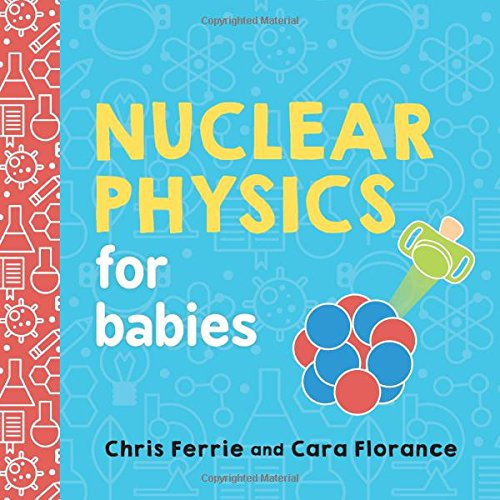Author: Chris Ferrie,Cara Florance
Published at: 01/05/2018
ISBN: 1492671177

Nuclear Physics for Babies gives simple explanations of complex ideas

This is a great book for kids as well as for adults. It has good and clear messages and pictures that make it excellent and readable for a little one. In this book, the author makes the complex thing easier by breaking down it. This is an interesting and informative book and makes complex science easy to understand.

Babies will learn

• The amazing process of nuclear decay
• Number of real concepts
• Illustration skills

This guide provides a perfect way to introduce basic concepts to all youngest scientists.

## 2. Nuclear Physics: A Very Short Introduction (Very Short Introductions)

In Nuclear Physics, Frank Close gives an account of how the area of physics has progressed, the recognition of how heavy nuclei are built up in the cores of stars and in supernovae, the identification of quarks and gluons, and the development of quantum chromodynamics (QCD). Some key concepts like the stability of different configurations of protons and neutrons in nuclei are explored in this book.

This book contains

• The fly in the cathedral
• Nuclear alchemy
• Powerful forces
• Nucleosynthesis
• Odds, evens, and shells
• Beyond the periodic table
• Exotic nuclei
• Applied nuclear physics.

## 3. Theoretical Nuclear Physics (Dover Books on Physics)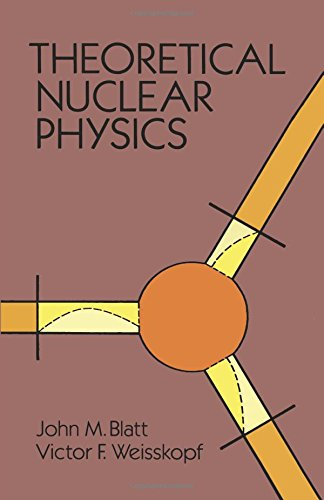Author: John M. Blatt,Victor F. Weisskopf
Published at: 18/10/2010
ISBN: 0486668274

This guide provides a good basis for specialists. A well-recommended book for the experimental physicist working in nuclear physics or graduate students who have had at least a one-term course in quantum mechanics and who know the essential concepts and problems of nuclear physics.

Some important topics are nuclear forces, nuclear spectroscopy, and two-, three- and four-body problems, explorations of nuclear reactions, beta-decay, and nuclear shell structure, etc.

Key coverage are

• General properties of the nucleus
• Two-body problems at low energies
• Nuclear forces
• Two-body problems at high energies
• Three and four body problems
• Nuclear spectroscopy
• Nuclear reactions: general theory
• Nuclear reactions: application of the experiments
• A formal theory of nuclear reactions
• Spontaneous decay of nuclei
• Interaction of nuclei with electromagnetic radiation
• Beta decay
• Nuclear shell structure.

## 4. Introductory Nuclear Physics

Introductory Nuclear Physics, written by Kenneth S. Krane is a great book containing basic concepts of nuclear structure, elements of quantum mechanics, nuclear properties, the force between nucleons, nuclear models, detecting nuclear radiation, alpha, beta and gamma decay, neutron physics, nuclear fission, nuclear fusion, nuclear spins and moment etc.

This book is divided into three units. Those are

• Basic nuclear structure
• Nuclear reactions

The writer did a good job of explaining things with proper explanations and equations.

## 5. Nuclear Physics in a Nutshell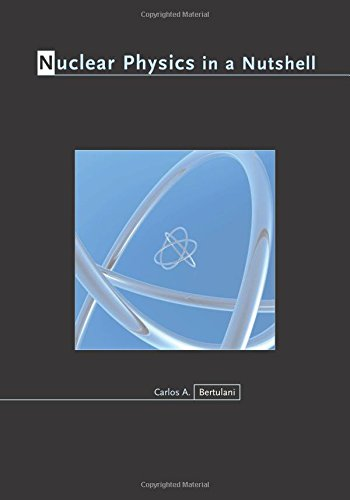Author: Carlos A. Bertulani
Published at: 23/04/2007
ISBN: 0691125058

This book contains huge content that helps you to learn many things at once. If you are a 3rd or 4th-year student or a graduate student and have some knowledge of quantum mechanics then Nuclear Physics, in a Nutshell, is the right book for you.

This book provides

• How simple models can provide an understanding of the properties of nuclei
• The nature of nuclear reactions
• Nuclear constituents and their characteristics
• Nuclear interactions
• Nuclear structure.

This book covers many important topics. Some of them are

• Quantum chromodynamics
• The two-nucleon system
• Electrostatic multipoles
• The magnetic moment with spin-orbit coupling
• Experimental data for the deuteron
• A square-well model for the deuteron
• The deuteron wavefunction
• Angular momentum coupling
• Two particles of spin
• Total wavefunction
• Particles in the continuum: scattering
• Partial wave expansion
• Low energy scattering
• Effective range theory
• Proton-proton scattering and much much more.

## 6. Physics in Nuclear MedicineAuthor: Simon R. Cherry PhD,James A. Sorenson PhD,Michael E. Phelps PhD
Published at: 26/04/2012
ISBN: 1416051988

Physics in Nuclear Medicine provides current, comprehensive guidance on the physics underlying modern nuclear medicine and imaging using radioactively labeled tracers. After reading this book you will be the master of the physics of nuclear medicine. Here you will find thorough explanations of analytic equations and illustrative graphs and get a better view of images and line art and find information more easily.

Key coverage are

• What is nuclear
• Basic atomic and nuclear physics
• Interaction of radiation with matter
• Electronic instrumentation for radiation detection systems
• Nuclear counting statistics
• Pulse-height spectrometry
• Problems in radiation detection and measurement
• Counting systems
• The gamma camera: basic principles
• The gamma camera: performance characteristics
• Image quality in nuclear medicine
• Tomographic reconstruction in nuclear medicine
• Single photon emission computed tomography
• Positron Emission Tomography
• Digital image processing in nuclear medicine.

## 7. Theoretical Nuclear And Subnuclear Physics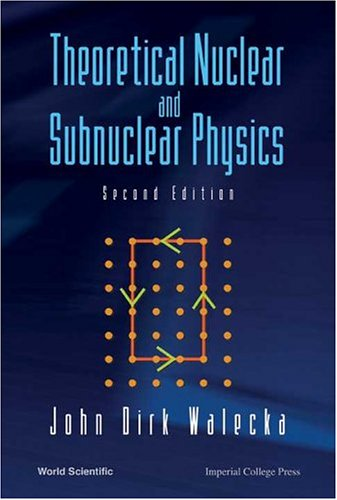Author: Senior Fellow Continuous Electron Beam Accelerator Facility (Cebaf) Governor's Distinguished Cebaf Professor John Dirk Walecka
Published at: 30/09/2004
ISBN: 9812388982

This is a very practical and technical book that advances your already existing nuclear physics knowledge. This book is recommended for the graduate student in physics or perhaps a physicist in another field who is curious about the current state of nuclear physics. It includes the history, experimental techniques, and theoretical formalism to help you learn the things properly.

It provides

• Modern nuclear theory
• Nonrelativistic many-body problem
• A standard model of the strong, electromagnetic, and weak interactions
• Basic nuclear structure
• The relativistic nuclear many-body problem
• Strong-coupling QCD
• Electroweak interactions with nuclei
• Many-particle shell model
• Effective field theory
• Density functional theory
• Heavy-ion reactions
• Quark-gluon plasma
• Neutrinos, and electron scattering.

## 8. Nuclear Physics

Nuclear Physics provides an in-depth look at the study of the atom and comprised of a series of Heisenberg’s lectures on the subject. This book is detailed and accessible enough for anyone interested in the subject.

This book gives you

• A short history of atomic physics
• The theory of the processes and reactions
• An unparalleled look at nuclear physics

W. Heisenberg was a German physicist who was interested in scientific and philosophical pursuits from a young age and established his career in the study of atomic and particle theory. He continuously contributed his research to the field of nuclear physics until his death.

## 9. Modern Atomic and Nuclear Physics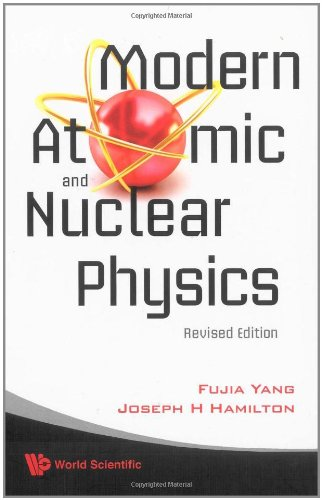Author: Joseph H Hamilton,Fujia Yang
Published at: 30/03/2010
ISBN: 9812836799

The writer of this book explains everything in a logical and easy to follow way. Each chapter contains a summary and problems that you will face during your study period.

Modern Atomic and Nuclear Physics gives students a basic understanding of

• Atoms
• Nucleus to leptons
• Quarks, and gluons
• The essential introductory quantum mechanics and special relativity

Some of the contents include the following

• The configuration of the atom: rutherford's model
• Quantum states of atoms: the Bohr model
• Fine structure in atomic spectra: electron spin
• Atoms containing many electrons: the Pauli exclusion
• X-Rays
• Introductory quantum mechanics I: concepts
• Introductory quantum mechanics II: the Schrödinger equation
• Basic concepts of nuclear physics.

## 10. Lectures on Nuclear Theory (Dover Books on Physics)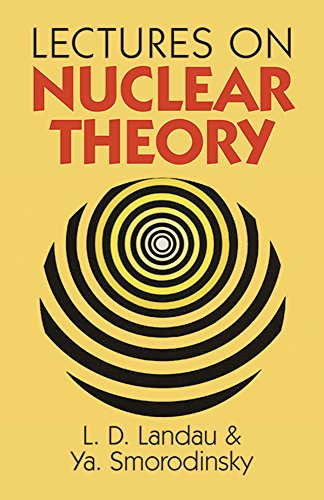Author: L. Landau,Ya. Smorodinsky
Published at: 19/07/2011
ISBN: 0486675130

This is a nice book on nuclear theory from which you can learn the basics. It gives you the most important underlying concepts of nuclear physics. This book is for the specialist in other fields who desires insight into the problems of nuclear physics and is must have for all physics graduate students

Covered topics are

• Nuclear forces
• The scattering of nucleons by nucleons and by high energies
• The independent particle model of nuclear structure
• The structure of the nucleus
• Light nuclei and heavy nuclei
• Nuclear reactions
• Statistical theory
• Optical model
• Deuteron reactions
• Pi-mesons, and interactions of pi-mesons with nucleons.

Thanks for reading this post. If you have any opinion don't hesitate to comment here. Also please subscribe our newsletter to get more updates.